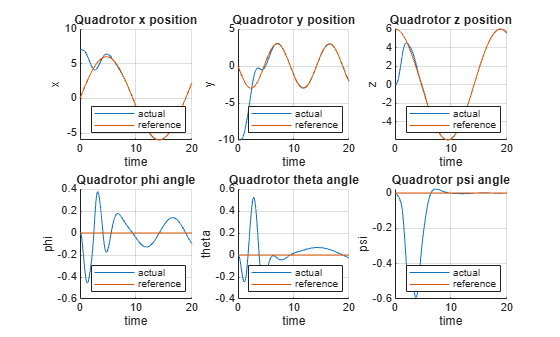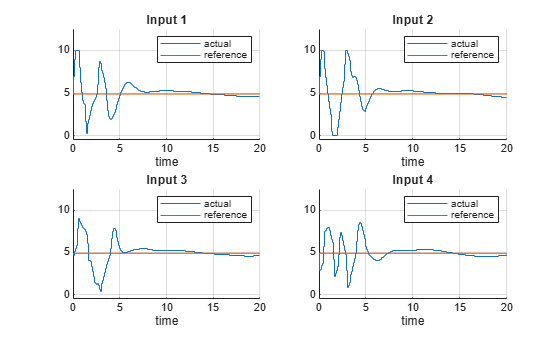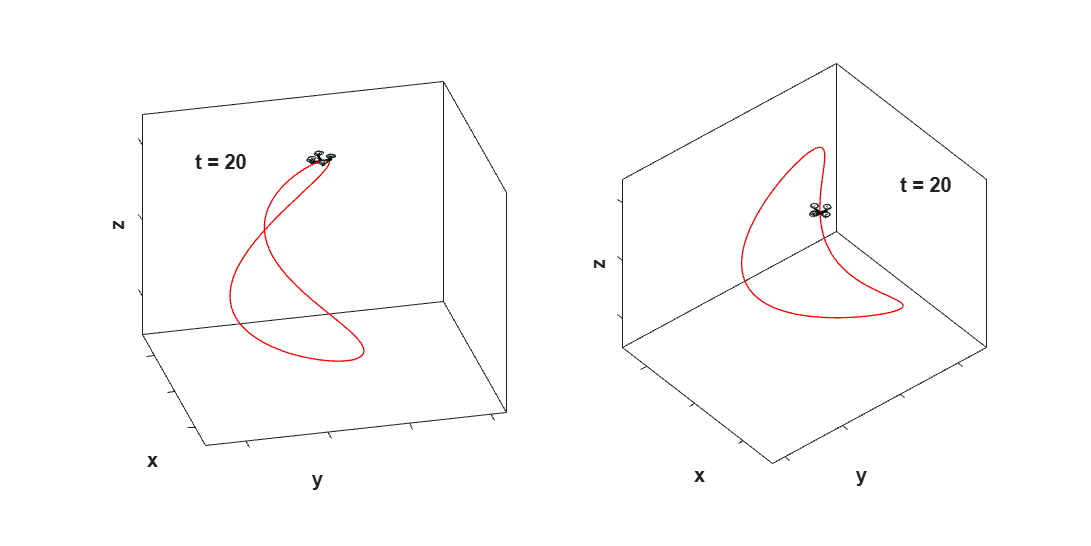# Control of Quadrotor Using Nonlinear Model Predictive Control

This example shows how to design a trajectory tracking controller for a quadrotor using nonlinear model predictive control (MPC).

The quadrotor has four rotors which are directed upwards. From the center of mass of the quadrotor, rotors are placed in a square formation with equal distance. The mathematical model for the quadrotor dynamics are derived from Euler-Lagrange equations .

The twelve states for the quadrotor are:

$\left[x,y,z,\varphi ,\theta ,\psi ,\underset{}{\overset{˙}{x}},\underset{}{\overset{˙}{y}},\underset{}{\overset{˙}{z}},\underset{}{\overset{˙}{\varphi }},\underset{}{\overset{˙}{\theta }},\underset{}{\overset{˙}{\psi }}\right]$,

where

• $\left[\mathit{x},\mathit{y},\mathit{z}\right]$denote the positions in the inertial frame

• Angle positions $\left[\varphi ,\theta ,\psi \right]$ are in the order of roll, pitch, and yaw

• The remaining states are the velocities of the positions and angles

The control inputs for the quadrotor are the squared angular velocities of the four rotors:

$\left[{\omega }_{1}^{2},{\omega }_{2}^{2},{\omega }_{3}^{2},{\omega }_{4}^{2}\right]$.

These control inputs create force, torque, and thrust in the direction of the body $\mathit{z}$-axis. In this example, every state is measurable, and the control inputs are constrained to be within [0,12] ${\left(\frac{\mathrm{rad}}{\mathit{s}}\right)}^{2}$.

The state function and state Jacobian function of the model are built and derived using Symbolic Math Toolbox™ software.

`getQuadrotorDynamicsAndJacobian;`
```ans = function_handle with value: @QuadrotorStateFcn ```
```ans = function_handle with value: @QuadrotorStateJacobianFcn ```

The `getQuadrotorDynamicsAndJacobian` script generates the following files:

• `QuadrotorStateFcn.m` — State function

• `QuadrotorStateJacobianFcn.m` — State Jacobian function

For details on either function, open the corresponding file.

### Design Nonlinear Model Predictive Controller

Create a nonlinear MPC object with 12 states, 12 outputs, and `4` inputs. By default, all the inputs are manipulated variables (MVs).

```nx = 12; ny = 12; nu = 4; nlobj = nlmpc(nx, ny, nu);```
```In standard cost function, zero weights are applied by default to one or more OVs because there are fewer MVs than OVs. ```

Specify the prediction model state function using the function name. You can also specify functions using a function handle.

`nlobj.Model.StateFcn = "QuadrotorStateFcn";`

Specify the Jacobian of the state function using a function handle. It is best practice to provide an analytical Jacobian for the prediction model. Doing so significantly improves simulation efficiency.

`nlobj.Jacobian.StateFcn = @QuadrotorStateJacobianFcn;`

```rng(0) validateFcns(nlobj,rand(nx,1),rand(nu,1));```
```Model.StateFcn is OK. Jacobian.StateFcn is OK. No output function specified. Assuming "y = x" in the prediction model. Analysis of user-provided model, cost, and constraint functions complete. ```

Specify a sample time of `0.1` seconds, prediction horizon of 18 steps, and control horizon of 2 steps.

```Ts = 0.1; p = 18; m = 2; nlobj.Ts = Ts; nlobj.PredictionHorizon = p; nlobj.ControlHorizon = m;```

Limit all four control inputs to be in the range [0,12].

`nlobj.MV = struct('Min',{0;0;0;0},'Max',{12;12;12;12});`

The default cost function in nonlinear MPC is a standard quadratic cost function suitable for reference tracking and disturbance rejection. In this example, the first 6 states $\left[x,y,z,\varphi ,\theta ,\psi \right]$ are required to follow a given reference trajectory. Because the number of MVs (4) is smaller than the number of reference output trajectories (6), there are not enough degrees of freedom to track the desired trajectories for all output variables (OVs).

`nlobj.Weights.OutputVariables = [1 1 1 1 1 1 0 0 0 0 0 0];`

In this example, MVs also have nominal targets to keep the quadrotor floating, which can lead to conflict between the MV and OV reference tracking goals. To prioritize targets, set the average MV tracking priority lower than the average OV tracking priority.

`nlobj.Weights.ManipulatedVariables = [0.1 0.1 0.1 0.1];`

Also, penalize aggressive control actions by specifying tuning weights for the MV rates of change.

`nlobj.Weights.ManipulatedVariablesRate = [0.1 0.1 0.1 0.1];`

### Closed-Loop Simulation

Simulate the system for 20 seconds with a target trajectory to follow.

```% Specify the initial conditions x = [7;-10;0;0;0;0;0;0;0;0;0;0]; % Nominal control that keeps the quadrotor floating nloptions = nlmpcmoveopt; nloptions.MVTarget = [4.9 4.9 4.9 4.9]; mv = nloptions.MVTarget;```

Simulate the closed-loop system using the `nlmpcmove` function, specifying simulation options using an `nlmpcmove` object.

```Duration = 20; hbar = waitbar(0,'Simulation Progress'); xHistory = x'; lastMV = mv; uHistory = lastMV; for k = 1:(Duration/Ts) % Set references for previewing t = linspace(k*Ts, (k+p-1)*Ts,p); yref = QuadrotorReferenceTrajectory(t); % Compute the control moves with reference previewing. xk = xHistory(k,:); [uk,nloptions,info] = nlmpcmove(nlobj,xk,lastMV,yref',[],nloptions); uHistory(k+1,:) = uk'; lastMV = uk; % Update states. ODEFUN = @(t,xk) QuadrotorStateFcn(xk,uk); [TOUT,YOUT] = ode45(ODEFUN,[0 Ts], xHistory(k,:)'); xHistory(k+1,:) = YOUT(end,:); waitbar(k*Ts/Duration,hbar); end close(hbar)```

### Visualization and Results

Plot the results, and compare the planned and actual closed-loop trajectories.

`plotQuadrotorTrajectory;`Because the number of MVs is smaller than the number of reference output trajectories, there are not enough degrees of freedom to track the desired trajectories for all OVs.

As shown in the figure for states $\left[x,y,z,\varphi ,\theta ,\psi \right]$ and control inputs,

• The states $\left[x,y,z\right]$ match the reference trajectory very closely within 7 seconds.

• The states $\left[\varphi ,\theta ,\psi \right]$ are driven to the neighborhood of zeros within 9 seconds.

• The control inputs are driven to the target value of 4.9 around 10 seconds.

You can animate the trajectory of the quadrotor. The quadrotor moves close to the "target" quadrotor which travels along the reference trajectory within 7 seconds. After that, the quadrotor follows closely the reference trajectory. The animation terminates at 20 seconds.

`animateQuadrotorTrajectory;`### Conclusion

This example shows how to design a nonlinear model predictive controller for trajectory tracking of a quadrotor. The dynamics and Jacobians of the quadrotor are derived using Symbolic Math Toolbox software. The quadrotor tracks the reference trajectory closely.

### References

 T. Luukkonen, Modelling and control of quadcopter, Independent research project in applied mathematics, Espoo: Aalto University, 2011.

 E. Tzorakoleftherakis, and T. D. Murphey. "Iterative sequential action control for stable, model-based control of nonlinear systems." IEEE Transactions on Automatic Control (2018).